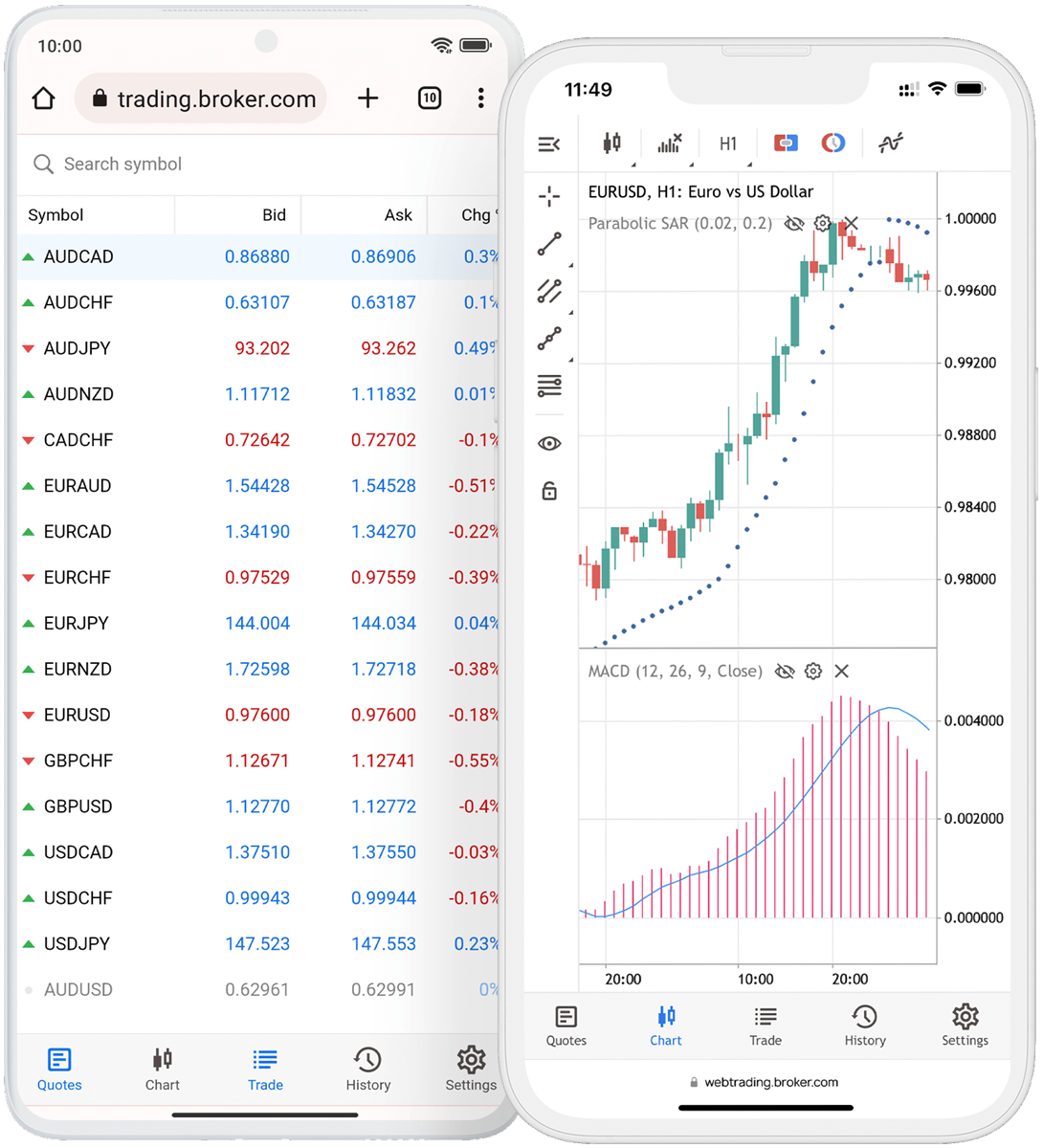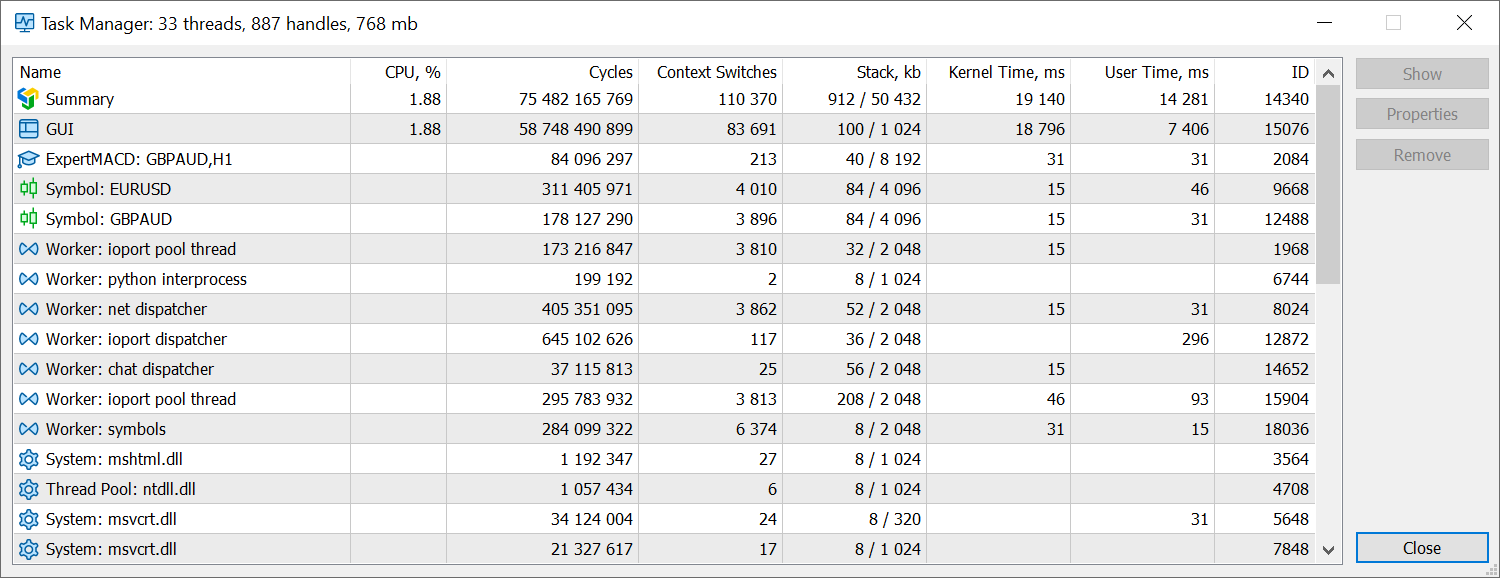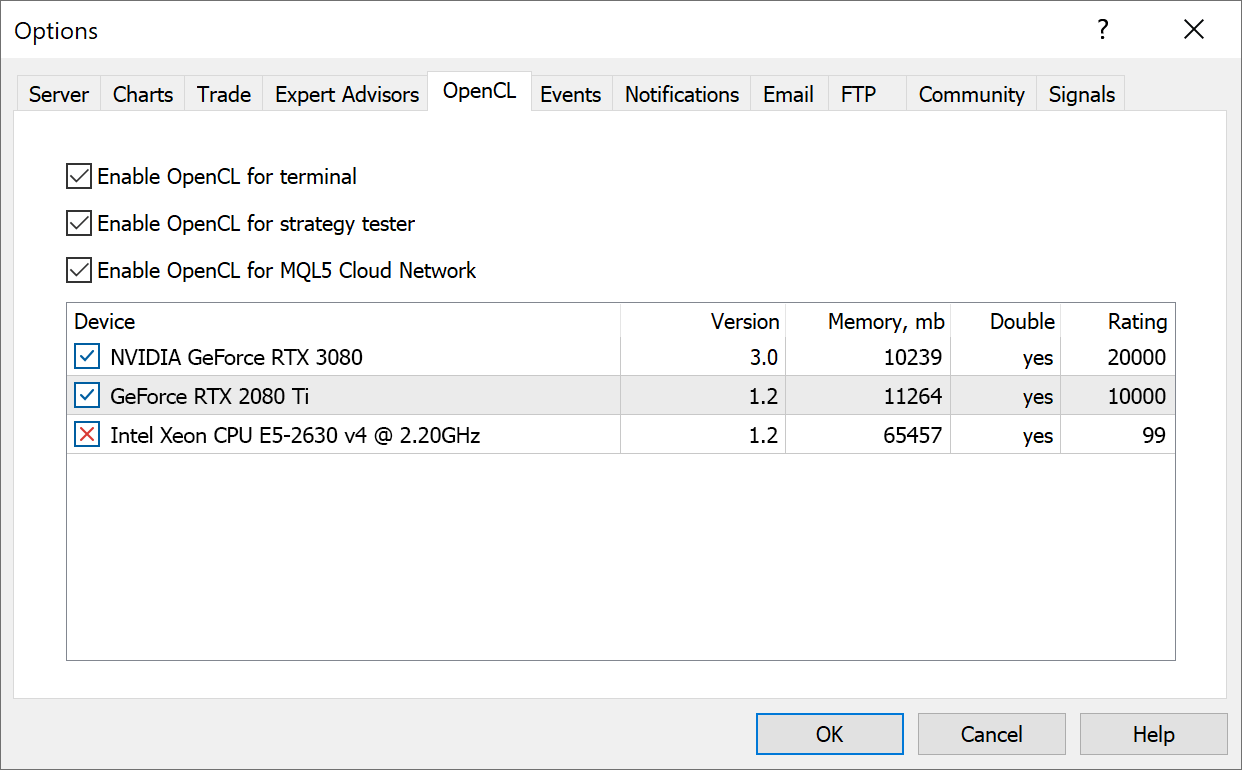# MetaTrader 5 build 3490: Mobile Web Terminal version and new matrix methods in MQL5

## What's new in MetaTrader 5

4 November 2022

Mobile version of the web platform

The new Web Terminal provides full-featured support for mobile devices. The interface will automatically adapt to the screen size, enabling efficient operations from iOS and Android phones and tablets:Also, the Web Terminal features a lot of fixes and improvements.

The new MetaTrader 5 Web Terminal supports the full set of trading functions. It enables users to:

• Work with demo and live accounts
• Receive any financial symbol quotes
• Analyze symbol quotes using more than 30 indicators and 20 graphical objects
• Use Economic Calendar data for fundamental analysis

### Terminal

1. Extended task manager features. The new version enables more accurate monitoring of consumed resources.

• Added display of the number of context switches.
• Added display of kernel mode operating time. An increase in this metric compared to the time spent in user mode can indicate system-level issues: drivers problems, hardware errors or slow hardware. For further details, please read the Microsoft Documentation.
• Added display of user mode operating time.2. New OpenCL tab in terminal settings for managing available devices. The new OpenCL manager enables explicit specification of devices to be used for calculations.3. Added indication of Stop Loss and Take Profit levels in the Depth of Market for accounts operating in FIFO mode (the mode can be enabled on the broker's side).

According to the FIFO rule, positions for each instrument can only be closed in the same order in which they were opened. To ensure FIFO-compliant position closing by stop levels, the following logic has been implemented on the client terminal side:

If multiple positions exist for the same instrument, the placing of stop levels for any of the positions causes the same levels to be placed for all other positions as well. Accordingly, if a level triggers, all positions will be closed in a FIFO-compliant order.

Now, when the user opens the Depth of Market for an instrument which already has open positions for, the levels of existing positions (if any) are automatically specified in the Stop Loss and Take Profit fields.

4. Fixed deletion of Stop Loss and Take Profit levels using X buttons in the Toolbox\Trade window. The error occurred when the quick trading function was disabled. A click on the button will open a trading dialog with an empty value of the relevant level.

5. Fixed graph captions and final commission calculations in the trading report. The section could show incorrect Profit in report statistics and incorrect values in Equity and Balance graph tooltips.

### MQL5

1. Added vector and matrix methods CopyTicks and CopyTicksRange. They enable easy copying of tick data arrays into vectors and matrices.
```bool matrix::CopyTicks(string symbol,uint flags,ulong from_msc,uint count);
bool vector::CopyTicks(string symbol,uint flags,ulong from_msc,uint count);

bool matrix::CopyTicksRange(string symbol,uint flags,ulong from_msc,ulong to_msc);
bool matrix::CopyTicksRange(string symbol,uint flags,ulong from_msc,ulong to_msc);```
The copied data type is specified in the 'flags' parameter using the ENUM_COPY_TICKS enumeration. The following values are available:
```COPY_TICKS_INFO    = 1,       // ticks resulting from Bid and/or Ask changes
COPY_TICKS_TRADE   = 2,       // ticks resulting from Last and Volume changes
COPY_TICKS_ALL     = 3,       // all ticks having changes
COPY_TICKS_TIME_MS = 1<<8,    // time in milliseconds
COPY_TICKS_BID     = 1<<9,    // Bid price
COPY_TICKS_LAST    = 1<<11,   // Last price
COPY_TICKS_VOLUME  = 1<<12,   // volume
COPY_TICKS_FLAGS   = 1<<13,   // tick flags```
If multiple data types are selected (only available for matrices), the order of the rows in the matrix will correspond to the order of values in the enumeration.

2. Expanded features of matrix::Assign and vector::Assign methods.

Now the matrix can be assigned a one-dimensional array or vector:
`bool matrix::Assign(const vector &vec);`
The result will be a one-row matrix.

Also, a matrix can now be assigned to a vector (matrix smoothing will be performed):
`bool vector::Assign(const matrix &mat);`
3. Added Swap methods for vectors and matrices.
```bool vector::Swap(vector &vec);
bool vector::Swap(matrix &vec);
bool vector::Swap(double &arr[]);
bool matrix::Swap(vector &vec);
bool matrix::Swap(matrix &vec);
bool matrix::Swap(double &arr[]);```
Each array, vector or matrix refers to a memory buffer which contains the elements of that object. The Swap method actually swaps pointers to these buffers without writing the elements to memory. Therefore, a matrix remains a matrix, and a vector remains a vector. Swapping a matrix and a vector will result in a one-row matrix with vector elements and a vector with matrix elements in a flat representation (see the Flat method).
```//+------------------------------------------------------------------+
//| Script program start function                                    |
//+------------------------------------------------------------------+
void OnStart()
{
//---
matrix a= {{1, 2, 3},

{4, 5, 6}};
Print("a before Swap: \n", a);
matrix b= {{5, 10, 15, 20},

{25, 30, 35, 40},

{45, 50, 55, 60}};
Print("b before Swap: \n", b);
//--- swap matrix pointers
a.Swap(b);
Print("a after Swap: \n", a);
Print("b after Swap: \n", b);
/*
a before Swap:
[[1,2,3]
[4,5,6]]
b before Swap:
[[5,10,15,20]
[25,30,35,40]
[45,50,55,60]]

a after Swap:
[[5,10,15,20]
[25,30,35,40]
[45,50,55,60]]
b after Swap:
[[1,2,3]
[4,5,6]]
*/
vector v=vector::Full(10, 7);
Print("v before Swap: \n", v);
Print("b before Swap: \n", b);
v.Swap(b);
Print("v after Swap: \n", v);
Print("b after Swap: \n", b);
/*
v before Swap:
[7,7,7,7,7,7,7,7,7,7]
b before Swap:
[[1,2,3]
[4,5,6]]

v after Swap:
[1,2,3,4,5,6]
b after Swap:
[[7,7,7,7,7,7,7,7,7,7]]
*/
}```
The Swap() method also enables operations with dynamic arrays (fixed-sized arrays cannot be passed as parameters). The array can be of any dimension but of an agreed size, which means that the total size of a matrix or vector must be a multiple of the array's zero dimension. The array's zero dimension is the number of elements contained at the first index. For example, for a dynamic three-dimensional array 'double array[]', the zero dimension is the product of the second and third dimension sizes: 2x3=6. So, such an array can only be used in the Swap method with matrices and vectors whose total size is a multiple of 6: 6, 12, 18, 24, etc.

Consider the following example:
```//+------------------------------------------------------------------+
//| Script program start function                                    |
//+------------------------------------------------------------------+
void OnStart()
{
//--- fill the 1x10 matrix with the value 7.0
matrix m= matrix::Full(1, 10, 7.0);
Print("matrix before Swap:\n", m);
//--- try to swap the matrix and the array
double array_small= {{1, 2, 3, 4, 5},

{6, 7, 8, 9, 10}};
Print("array_small before Swap:");
ArrayPrint(array_small);
if(m.Swap(array_small))
{
Print("array_small after Swap:");
ArrayPrint(array_small);
Print("matrix after Swap: \n", m);
}
else // the matrix size is not a multiple of the first array dimension
{
Print("m.Swap(array_small) failed. Error ", GetLastError());
}
/*
matrix before Swap:
[[7,7,7,7,7,7,7,7,7,7]]
array_small before Swap:
[,0]     [,1]     [,2]     [,3]     [,4]
[0,]  1.00000  2.00000  3.00000  4.00000  5.00000
[1,]  6.00000  7.00000  8.00000  9.00000 10.00000
m.Swap(array_small) failed. Error 4006
*/
//--- use a larger matrix and retry the swap operation
double array_static= {{1, 2, 3, 4, 5, 6, 7, 8, 9, 10},

{2, 4, 6, 8, 10, 12, 14, 16, 18, 20},

{3, 6, 9, 12, 15, 18, 21, 24, 27, 30}
};
Print("array_static before Swap:");
ArrayPrint(array_static);
if(m.Swap(array_static))
{
Print("array_static after Swap:");
ArrayPrint(array_static);
Print("matrix after Swap: \n", m);
}
else // a static array cannot be used to swap with a matrix
{
Print("m.Swap(array_static) failed. Error ", GetLastError());
}
/*
array_static before Swap:
[,0]     [,1]     [,2]     [,3]     [,4]     [,5]     [,6]     [,7]     [,8]     [,9]
[0,]  1.00000  2.00000  3.00000  4.00000  5.00000  6.00000  7.00000  8.00000  9.00000 10.00000
[1,]  2.00000  4.00000  6.00000  8.00000 10.00000 12.00000 14.00000 16.00000 18.00000 20.00000
[2,]  3.00000  6.00000  9.00000 12.00000 15.00000 18.00000 21.00000 24.00000 27.00000 30.00000
m.Swap(array_static) failed. Error 4006
*/
//--- another attempt to swap an array and a matrix
double array_dynamic[];    // dynamic array
ArrayResize(array_dynamic, 3); // set the first dimension size
ArrayCopy(array_dynamic, array_static);
//--- now use a dynamic array for swap
if(m.Swap(array_dynamic))
{
Print("array_dynamic after Swap:");
ArrayPrint(array_dynamic);
Print("matrix after Swap: \n", m);
}
else //  no error
{
Print("m.Swap(array_dynamic) failed. Error ", GetLastError());
}
/*
array_dynamic after Swap:
[,0]    [,1]    [,2]    [,3]    [,4]    [,5]    [,6]    [,7]    [,8]    [,9]
[0,] 7.00000 7.00000 7.00000 7.00000 7.00000 7.00000 7.00000 7.00000 7.00000 7.00000
matrix after Swap:
[[1,2,3,4,5,6,7,8,9,10,2,4,6,8,10,12,14,16,18,20,3,6,9,12,15,18,21,24,27,30]]
*/
}```
4. Added LossGradient method for vectors and matrices. This method calculates a vector or matrix of partial derivatives of the loss function on predicted values. In linear algebra, such a vector is referred to as a gradient and is used in machine learning.
```vector vector::LossGradient(const vector &expected,ENUM_LOSS_FUNCTION loss) const;
matrix matrix::LossGradient(const matrix &expected,ENUM_LOSS_FUNCTION loss) const;```
5. Enabled use of FOREIGN KEYS in SQLite to enforce relationships between tables in SQL queries.   Example:
```CREATE TABLE artist(
artistid    INTEGER PRIMARY KEY,
artistname  TEXT
);

CREATE TABLE track(
trackid     INTEGER,
trackname   TEXT,
trackartist INTEGER,
FOREIGN KEY(trackartist) REFERENCES artist(artistid)
);```

6. Fixed selection of the appropriate class method depending on the method and object constness.

### MetaEditor

1. Increased allowable length of comments in commits to MQL5 Storage. Detailed comments when committing changes to the repository are considered good practice when working in large projects, but previously the comment length has been limited to 128 characters. The allowed length is now up to 260 characters.

### MetaTester

1. Increased sensitivity of the testing speed switch in visual mode.

Fixed errors reported in crash logs.